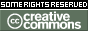Please use this identifier to cite or link to this item: http://bura.brunel.ac.uk/handle/2438/25870
 Title: Largest nearest-neighbour link and connectivity threshold in a polytopal random sample Authors: Penrose, MDYang, X Keywords: probability;math.PR;60D05;60F15;05C80 Issue Date: 6-Jan-2023 Publisher: Cornell University Citation: Penrose, M.D. and Yang, X. (2023) 'Largest nearest-neighbour link and connectivity threshold in a polytopal random sample', arXiv:2301.02506v1 [math.PR], pp. 1 - 26. doi: 10.48550/arXiv.2301.02506. Abstract: Copyright © 2023 The Authors. Let $X_1,X_2, \ldots$ be independent identically distributed random points in a convex polytopal domain $A \subset \mathbb{R}^d$. Define the largest nearest neighbour link $L_n$ to be the smallest $r$ such that every point of $\mathcal X_n:=\{X_1,\ldots,X_n\}$ has another such point within distance $r$. We obtain a strong law of large numbers for $L_n$ in the large-$n$ limit. A related threshold, the connectivity threshold $M_n$, is the smallest $r$ such that the random geometric graph $G(\mathcal X_n, r)$ is connected. We show that as $n \to \infty$, almost surely $nL_n^d/\log n$ tends to a limit that depends on the geometry of $A$, and $nM_n^d/\log n$ tends to the same limit. Description: Material is not peer-reviewed by arXiv - the contents of arXiv submissions are wholly the responsibility of the submitter and are presented “as is” without any warranty or guarantee. By hosting works and other materials on this site, arXiv, Cornell University, and their agents do not in any way convey implied approval of the assumptions, methods, results, or conclusions of the work. URI: https://bura.brunel.ac.uk/handle/2438/25870 DOI: https://doi.org/10.48550/arXiv.2301.02506 Other Identifiers: ORCID iD: Xiaochuan Yang https://orcid.org/0000-0003-2435-4615https://arxiv.org/abs/2301.02506v1 Appears in Collections: Dept of Mathematics Research Papers

Files in This Item:
File Description SizeFormat
This item is licensed under a Creative Commons License